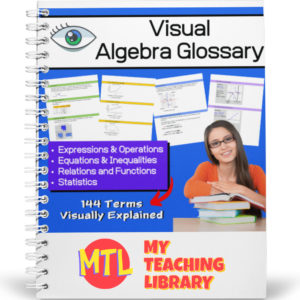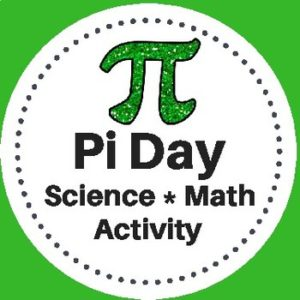Showing 1–20 of 25 results

• Sale!\$7.00 \$5.50

This consumer math bundle will teach students about both simple and compound interest and give them the practice they need to help them master solving word problems!

Includes:

•\$1.50

Students will complete a crossword puzzle by answering compound interest word problems. Also included, an optional number search puzzle which will provide an answer bank to use when completing the crossword puzzle! Answer Keys provided.

Sample problem: How much interest does a \$472 investment earn at 7% compounded annually over eight years?

•\$1.50

Students will complete a crossword puzzle by answering simple interest word problems. Also included, an optional number search puzzle which will provide an answer bank to use when completing the crossword puzzle! Answer Keys provided.

Sample problem: If you put \$5.62 in a saving account that pays 3% for eight years, what is the amount of money you will have at the end of the eight years?

•\$4.00

This consumer math resource has been designed to help teach students and give them the practice they need to master the concepts taught.

The first two pages will explain interest (simple and compound) and give students the formulas they will need to solve the word problems. There are 60 word problems in all. 3 sets of 10 simple interest problems and 3 sets of 10 compound interest problems. Answer keys are provided.

•\$3.50

Give students the practice they need when learning to solve one-step, one-variable equations! This resource provides 7 different worksheets, each with 25 problems for a total of 175 problems. All equations in this set of worksheets use only the properties of addition and subtraction.

•\$15.00

Give your Algebra I and Algebra II students a tool they can really use! This Visual Algebra Glossary will be an invaluable resource giving them explanations, definitions and examples of 144 terms. Easy to follow and understand, students will turn to this resource while learning new concepts, completing work and studying for exams.

There are four color coded sections, each with an term index:

• – Expressions & Operations
• – Equations & Inequalities
• – Relations & Functions
• – Statistics

73 pages

•\$1.50

Help students learn and remember the difference between regular and irregular shapes by displaying this classroom poster!

•\$3.00

This resource is designed to give students the practice the need to become proficient at multiplying decimals. There are three levels, 15 worksheets (16 problems each) and answer keys. That’s 240 problems – EACH WORKSHEET includes a different growth mindset quote!
(See description below for more details)

•\$3.00

This resource is designed to give students the practice the need to become proficient at multiplying decimal numbers. There are three levels, 15 worksheets (20 problems each) and answer keys. That’s 300 problems! Use as practice sheets, homework, or for quizzes and tests.

Also included: Visual poster to help students with ‘where to place’ the decimal point in their answers.

3 Levels:

• Level 1 (5 worksheets)
Tenths multiplied by tenths
• Level 2 (5 worksheets)
Tenths multiplied by hundreds and/or by thousandths
Hundredths multiplied by hundredths and/or by thousandths
• Level 3 (5 worksheets)
Mixed from tenths/hundredths/thousandths multiplied by tenths/hundredths/thousandths
•Easy to use, just print and go…This Vocabulary Terms Definitions Worksheet resource provides a template for students to use time and time again with any subject for any vocabulary that you are asking them to study.

Each page provides space for 5 words. Students will write each terms to be studied and a definition. (Option: If appropriate to the lesson(s), you can assign students to write synonyms or antonyms on the last line as there is plenty of space to do that!)

Use for Language Arts, Science, Social Studies, Math – ANY SUBJECT!

•\$5.00

Complete Solar System Science – Math Unit designed to help middle school students understand and compare the sizes of the parts of our solar system and how they compare. This unit will take at least 2 weeks to complete.

Included: Teacher’s Guide, Student Workbook, Answer Keys

•\$3.00

This Pi Day Activity has been designed for Science and Math classrooms. Students will use measuring tools to determine the radius, diameter, and circumference of various circular objects. They will then use the data that they collect to calculate pi.

*This activity gives students the opportunity to clearly understand the concept of the number pi and practice math skills through a hands-on activity with a variety of circular objects.

•\$1.25

Studying Roman Numerals? Display this colorful poster on a bulletin board or in a Math center! Includes roman numerals for 1, 5, 10, 50, 100, 500 and 1,000.

•\$3.00
Here are 3 colorful “Geometry Gem” posters to display in your classroom or to place in a math, learning center as a reference.
See description below for a list of what is included!
•\$3.00

Help your students reinforce their knowledge and Math skills with these 15 worksheets (210 problems) on dividing fractions! Students will divide fractions (numerator of 1) by whole numbers and whole numbers by fractions (numerator of 1).

•\$5.00

121 Math Posters – Each displaying a math term and explanation!

Designed for 4th – 12th Grades

•\$1.00

This resource, Types of Angles – Classroom Poster Set, includes 4 posters for your Math classroom…

1. Obtuse Angle
2. Acute Angle
3. Straight Angle
4. Right Angle

Print and for years of use, laminate!

•\$1.25

Here are three Math Polygon Family Tree posters to display on a bulletin board or you your Math centers. These will be a great visual addition to help introduce the relationships between shapes and shape vocabulary.

Included:

• Poster #1 Polygons will show: triangle pentagon, heptagon, quadrilateral, hexagon, octagon,
• Poster #2 Triangles will show: acute (scalene, isosceles, equilateral), obtuse (scalene, isosceles), right (scalene, isosceles)
• Poster #3 Quadrilaterals will show parallelograms (rhombuses, rectangles, squares), non-parallelograms (trapezoids, trapeziums, kites)
•\$3.50

This unit has been designed to help your students learn to identify, create, add and subtract roman numerals. Includes 387 problems (13 worksheets plus a crossword puzzle).

Students will be converting numbers and number words to roman numerals and visa versa. They will also be given addition and subtraction problems (using roman numerals) to solve.

Includes:

• – 2 pgs of instructional text
• – flash cards
• – 13 worksheets
• – 1 crossword puzzle
•